Custom math worksheets at your fingertips# Math worksheet template "Binomic2"

This is not a ready-to-download math worksheet. It is a template, that is a preconfgured list of problems with parameters, that you can use to create your own math worksheet from. You can then change the resulting worksheet if some of the parameters need any tweaking. To use this template and create your own custom worksheet, you have to register first so you can edit worksheets. You can register and create an account in a minute - there is a free trial available. Register here, and you can use this template. There is no charge for joining and as a new member, you will get a free amount of starting credits. After registering, please choose the tab "My worksheets", then select "View templates".

## Description

Use the binomic formulas to simplify and expand terms.

## Elaboration

This worksheet deals with advanced exercises on binomic formulas. The first task is to use binomic formulas in a simple application to multiply and simplify terms. The second task combines the binomic formulas with other simple terms. In addition to using the binomic formula to expand the binomic formulas when simplifying terms, the other terms must also be integrated to simplify the term. Two further tasks follow, in which the opposite approach is to be taken: A given term contains the multiplied expression of a binomic formula as a partial term. This must be recognized and taken into account in the required factorization. This worksheet concludes with two exercises in which the elements of binomic formulas must be recognised and written down in tabular form. The tables contain various presettings. One table deals with the first two binomic formulas, another with the third binomic formula.

## Preview

This is what the worksheet will look like. On the left hand side, the problem type is shown with its quickname. On the right, you will see a preview of the problem. This is just a sample, as dw-math will generate a new one when you load the template. Click on the underlined problem name to jump to the problem's detail page. You can download preview worksheets and solution sheets for this problem there. Note that all problem numbers are show as one here, in the worksheet they will be numbered sequentially. The worksheet's header and footer are not shown here.

 Expand and simplify binomic formula, advancedApply binomic formula expansion rules to simplify term. Advanced version mit multiples of variables as summands.Quickname: 7517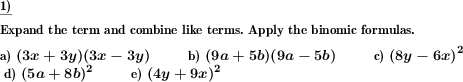Simplify term with binomic formula, combine like termsSimplify a term which contains a binomic formulaQuickname: 1444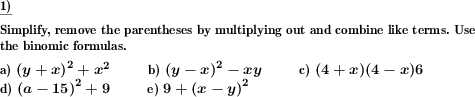Extract binomic formula from termA binomic formula is hidden in a term. Factor out the binomic formular or extract the summand.Quickname: 1825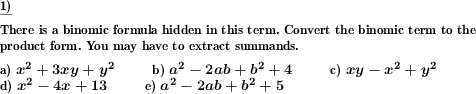Extract binomic formula from termA binomic formula is hidden in a term. Factor out the binomic formular or extract the summand.Quickname: 1825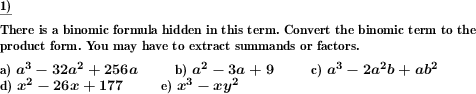Examine the elements of binomic formulasElements of a binomic formula and their expanded form are grouped in a table. Fill the empty spaces.Quickname: 7596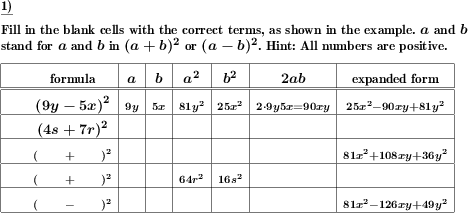Examine the elements of binomic formulasElements of a binomic formula and their expanded form are grouped in a table. Fill the empty spaces.Quickname: 7596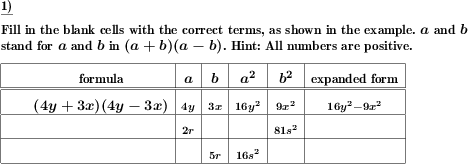These informational pages with samples describe math problems that can be combined on custom math worksheets with solutions for home and K-12 school use.
Deutsche Seiten
×